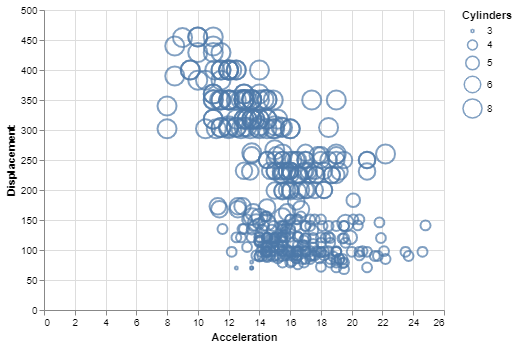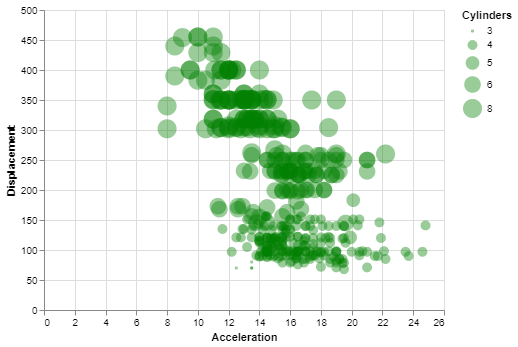Related Articles
How To Make Bubble plot with Altair in Python?
• Last Updated : 26 Nov, 2020

Prerequisite: Introduction to Altair in Python

Altair is a simple and easy to use statistical visualization library for python. It contains many types of built-in plots and various options to modify the properties and generate other plots. Bubble Plot is a very useful visualization for bivariate analysis of data with respect to a third variable. It is not readily available in the Altair library but can be made by doing some simple modifications to the scatter plot.

## What is a Bubble Plot?

Bubble Plot is basically a scatter plot between two variables/data columns where in place of the data points, there are bubbles/circles of varying sizes indicating the third variable. The third variable can be of a quantitative, ordinal, or nominal type, but the best type to be used in bubble plot is the ordinal type, i.e. data having a specific ordering. The legend shows which circle size corresponds to which data value.

A bubble plot can help us see the relationship between two variables with respect to a third variable. The bigger the bubble, the bigger value of data it corresponds to.

## Creating a Bubble Plot

To make a bubble plot, the user simply has to map a suitable variable from the dataset to the size encoding in a simple scatter plot.

The datasets used in these articles are from the Vega_datasets library.

## Python3

 `# Python3 program to illustrate ``# How to make a bubble plot``# using the altair library``   ` `# Importing altair and vega_datasets ``import` `altair as alt ``from` `vega_datasets ``import` `data ``   ` `# Selecting the cars dataset ``cars ``=` `data.cars() ``   ` `# Making the base scatter plot ``alt.Chart(cars).mark_point().encode( ``   ` `  ``# Map the sepalLength to x-axis ``    ``x ``=` `'Acceleration'``, ``   ` `  ``# Map the petalLength to y-axis ``    ``y ``=` `'Displacement'``,``   ` `  ``# Map the Cylinders variable to size``  ``# and specify it as a nominal variable``      ``size ``=` `'Cylinders:N'``)`

Output:Simple Bubble Plot using Altair

### Customizing the Bubble Plot

You can do the following customizations to the bubble plot:

• Color: You can change the default color of the bubbles by setting the color parameter of the mark_point() method.
• Opacity: You can change the default opacity of the bubbles by setting the opacity parameter of the mark_point() method. It ranges from 0 to 1.
• Filled: This is false by default, but you can change the filled parameter to true, thereby filling the bubble with the specified color.

Example:

## Python3

 `# Python3 program to illustrate``# how to customize a bubble plot`` ` `# Importing altair and vega_datasets``import` `altair as alt``from` `vega_datasets ``import` `data`` ` `# Selecting the cars dataset``cars ``=` `data.cars()`` ` `# Making the base scatter plot``# and adding the customizations``alt.Chart(cars).mark_point(color``=``'green'``,``                           ``filled``=``True``,``                           ``opacity``=``0.4``).encode(``   ` `    ``# Map the sepalLength to x-axis``    ``x``=``'Acceleration'``,``   ` `    ``# Map the petalLength to y-axis``    ``y``=``'Displacement'``,``   ` `    ``# Map the Cylinders variable to size``    ``# and specify it as a nominal variable``    ``size``=``'Cylinders:N'``)`

Output:Customized Bubble Plot using Altair

Attention geek! Strengthen your foundations with the Python Programming Foundation Course and learn the basics.

To begin with, your interview preparations Enhance your Data Structures concepts with the Python DS Course. And to begin with your Machine Learning Journey, join the Machine Learning – Basic Level Course

My Personal Notes arrow_drop_up